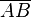# Distance facts for kids

Kids Encyclopedia Facts

Distance is how far one thing is from another thing. It is also a measure of the space between two things. It can be measured along any path. Thus, someone who goes around in a circle has traveled a distance, even though his position has not changed.

In geometry, the distance between two points A and B is sometimes written as$\overline{AB}$. Pythagorean theorem is often used in the calculation of distance. Distance is a scalar, and thus is different from displacement. Displacement is a vector that measures distance with a straight line (and in only one path). Displacement is the shortest way to travel the distance.

## Examples

• One ball is 5 feet from another ball. The distance between the two balls is 5 feet.
• John walks at a speed of 6 kilometres per hour for one hour. John walks a distance of 6 kilometres.
• A circle is a curved line. Each point on the circle is the same distance from the centre of the circle.

## Images for kidsDistance Facts for Kids. Kiddle Encyclopedia.